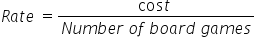Mathematics
Easy

Question

# At the toy store we get 2 board games for \$8.8. The cost of 5 board games will be _______________.

## 15192216Hint:

## The correct answer is: 22

### Cost of 2 board games is \$8.8.We have to find the rate. We will find it by taking the ratio of the cost of board games and number of board games. As the rate is constant, we will use this value to find cost of 5 board games.So the equation becomeSo the rate = \$4.4 per board gameLet’s find the cost of 5 board games.We will rearrange this for cost.For this we will multiply both sides by ‘number of board games’.Rate × number of board games = costCost = Rate × number of board gamesSubstituting the values we have foundCost = 4.4 × 5Cost = \$22Therefore, cost of 5 board games is \$22.

We have to be careful about units. Same quantities must have same unit.

### Related Questions to study#### With Turito Foundation.#### Get an Expert Advice From Turito.